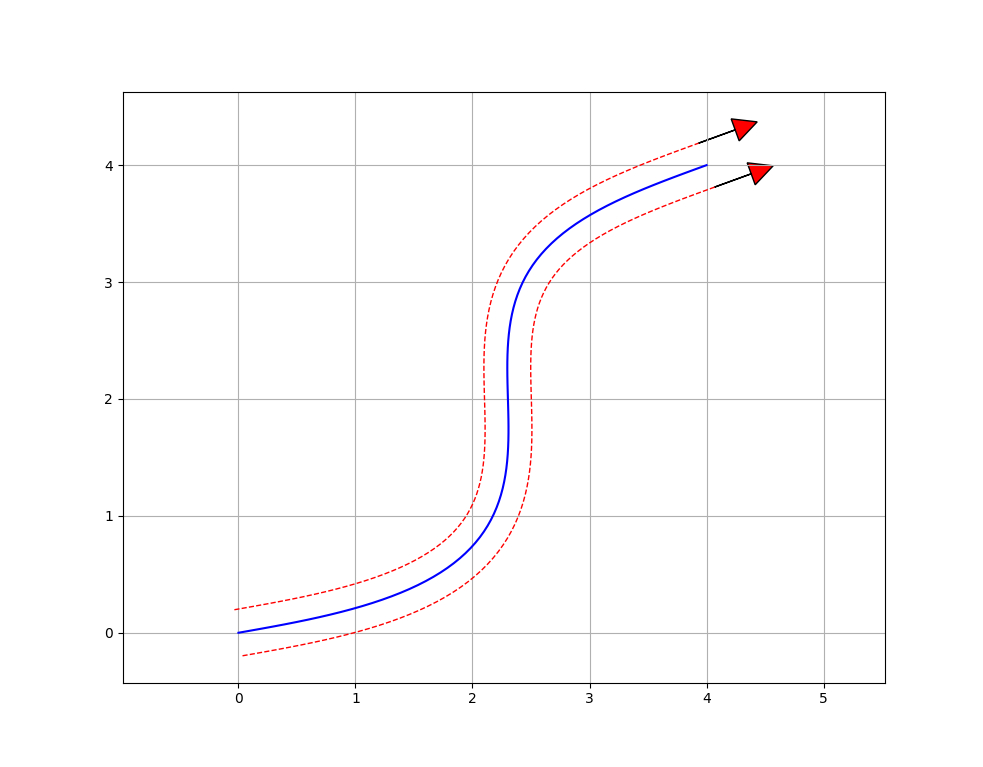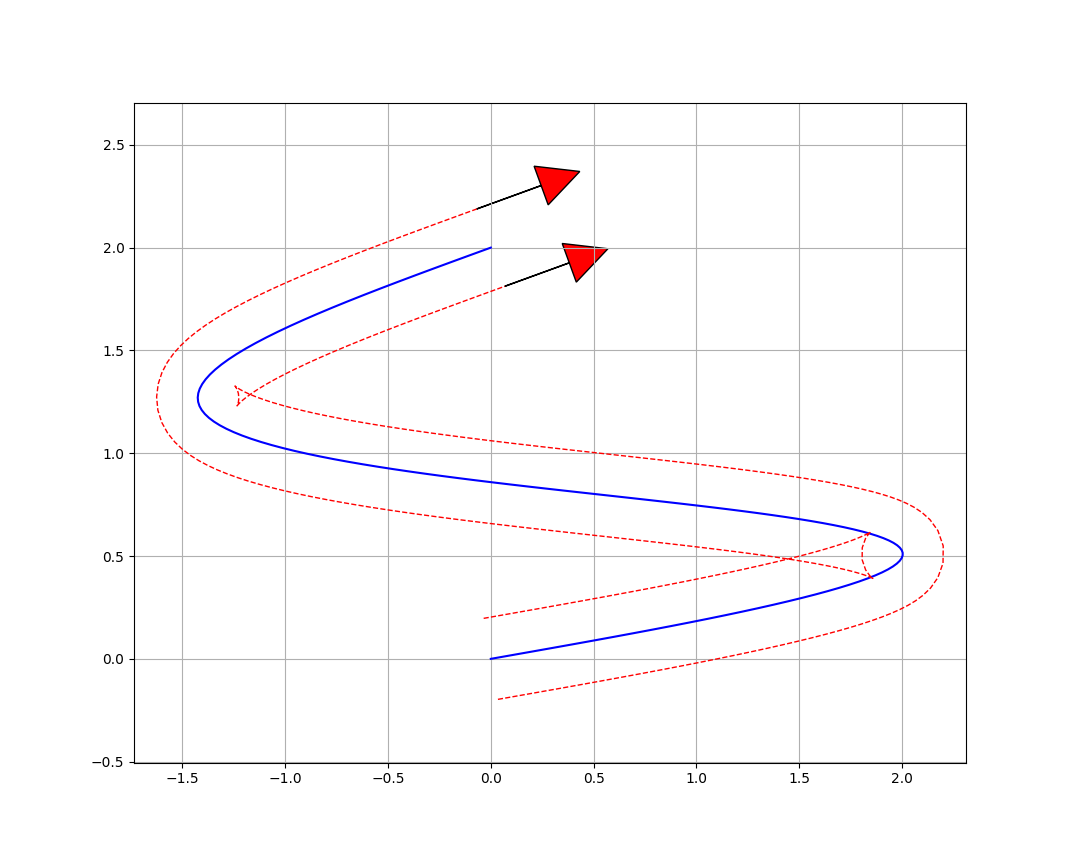# Example Paths¶

## Basic Path¶The above path can be created in three simple steps. First, define the Constraints with the robot’s maximum velocity, acceleration, and jerk when driving:

#include "squiggles.hpp"

const double MAX_VEL   = 2.0; // in meters per second
const double MAX_ACCEL = 3.0; // in meters per second per second
const double MAX_JERK  = 6.0; // in meters per second per second per second

squiggles::Constraints constraints = squiggles::Constraints(MAX_VEL, MAX_ACCEL, MAX_JERK);


Then measure the width between the robot’s wheels and create a SplineGenerator with the constraints and the width measurement:

#include "squiggles.hpp"

const double MAX_VEL     = 2.0; // in meters per second
const double MAX_ACCEL   = 3.0; // in meters per second per second
const double MAX_JERK    = 6.0; // in meters per second per second per second
const double ROBOT_WIDTH = 0.4; // in meters

squiggles::Constraints constraints = squiggles::Constraints(MAX_VEL, MAX_ACCEL, MAX_JERK);
squiggles::SplineGenerator generator = squiggles::SplineGenerator(
constraints,
std::make_shared<squiggles::TankModel>(ROBOT_WIDTH, constraints));


And finally let’s set the starting and ending poses as shown in the image above:

#include "squiggles.hpp"

const double MAX_VEL     = 2.0; // in meters per second
const double MAX_ACCEL   = 3.0; // in meters per second per second
const double MAX_JERK    = 6.0; // in meters per second per second per second
const double ROBOT_WIDTH = 0.4; // in meters

squiggles::Constraints constraints = squiggles::Constraints(MAX_VEL, MAX_ACCEL, MAX_JERK);
squiggles::SplineGenerator generator = squiggles::SplineGenerator(
constraints,
std::make_shared<squiggles::TankModel>(ROBOT_WIDTH, constraints));

std::vector<squiggles::ProfilePoint> path = generator.generate({
squiggles::Pose(0.0, 0.0, 1.0),
squiggles::Pose(4.0, 4.0, 1.0)});


## Tight Path¶The generated paths can get a bit more interesting when trying to make tight turns. The path shown above sends negative velocities to the inner wheel during the turns in order to make turns in a small space.

We can reuse the SplineGenerator from the previous section for this second path. It is often easiest, though not required, to create the SplineGenerator once and call its generate() method as many times as needed.

std::vector<squiggles::ProfilePoint> path = generator.generate({
squiggles::Pose(0.0, 0.0, 1.0),
squiggles::Pose(0.0, 2.0, 1.0)});